# JP6375618B2 - Electrostatic protection circuit and semiconductor integrated circuit device - Google Patents

## Info

Publication number
JP6375618B2
JP6375618B2 JP2013253931A JP2013253931A JP6375618B2 JP 6375618 B2 JP6375618 B2 JP 6375618B2 JP 2013253931 A JP2013253931 A JP 2013253931A JP 2013253931 A JP2013253931 A JP 2013253931A JP 6375618 B2 JP6375618 B2 JP 6375618B2
Authority
JP
Japan
Prior art keywords
node
voltage
transistor
circuit
electrostatic protection
Prior art date
Legal status (The legal status is an assumption and is not a legal conclusion. Google has not performed a legal analysis and makes no representation as to the accuracy of the status listed.)
Active
Application number
JP2013253931A
Other languages
Japanese (ja)
Other versions
JP2015115339A (en
JP2015115339A5 (en
Inventor

Original Assignee
セイコーエプソン株式会社
Priority date (The priority date is an assumption and is not a legal conclusion. Google has not performed a legal analysis and makes no representation as to the accuracy of the date listed.)
Filing date
Publication date
Application filed by セイコーエプソン株式会社 filed Critical セイコーエプソン株式会社
Priority to JP2013253931A priority Critical patent/JP6375618B2/en
Publication of JP2015115339A publication Critical patent/JP2015115339A/en
Publication of JP2015115339A5 publication Critical patent/JP2015115339A5/ja
Application granted granted Critical
Publication of JP6375618B2 publication Critical patent/JP6375618B2/en
Active legal-status Critical Current
Anticipated expiration legal-status Critical

• 239000004065 semiconductors Substances 0.000 title claims description 38
• 239000003990 capacitor Substances 0.000 claims description 26
• 230000003068 static Effects 0.000 claims description 10
• 238000010586 diagrams Methods 0.000 description 30
• 230000015556 catabolic process Effects 0.000 description 11
• 230000000052 comparative effects Effects 0.000 description 8
• 230000001681 protective Effects 0.000 description 6
• 230000000694 effects Effects 0.000 description 5
• 238000004519 manufacturing process Methods 0.000 description 5
• 230000001629 suppression Effects 0.000 description 5
• 239000004676 acrylonitrile butadiene styrene Substances 0.000 description 3
• 238000000034 methods Methods 0.000 description 3
• 230000002633 protecting Effects 0.000 description 3
• 229910044991 metal oxides Inorganic materials 0.000 description 2
• 150000004706 metal oxides Chemical class 0.000 description 2
• 239000002184 metals Substances 0.000 description 2
• 230000000630 rising Effects 0.000 description 2
• 238000004088 simulation Methods 0.000 description 2
• 239000000758 substrates Substances 0.000 description 2
• 241000287181 Sturnus vulgaris Species 0.000 description 1
• 230000036887 VSS Effects 0.000 description 1
• 230000001276 controlling effects Effects 0.000 description 1
• 230000000875 corresponding Effects 0.000 description 1
• 239000010410 layers Substances 0.000 description 1
• 230000004048 modification Effects 0.000 description 1
• 238000006011 modification reactions Methods 0.000 description 1

## Images

•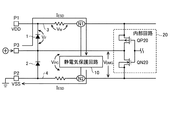•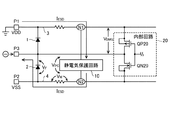•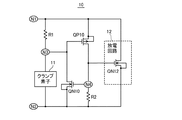•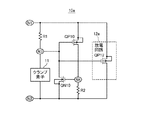•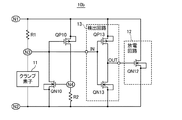•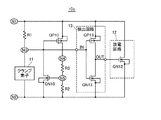•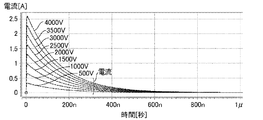•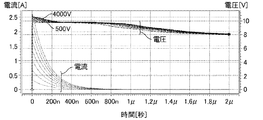•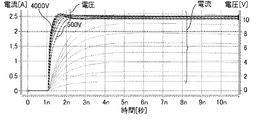•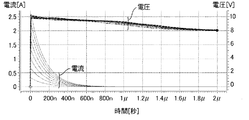•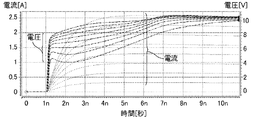•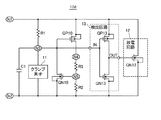•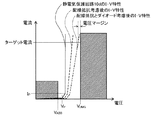•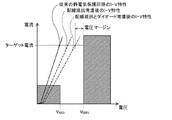•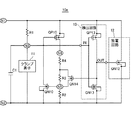•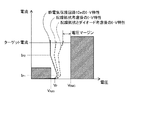•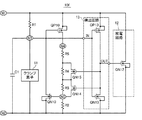•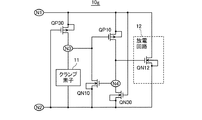•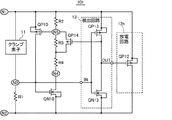•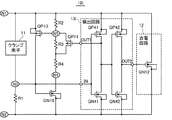•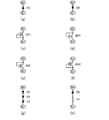•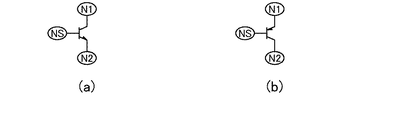## Classifications

• HELECTRICITY
• H02GENERATION; CONVERSION OR DISTRIBUTION OF ELECTRIC POWER
• H02HEMERGENCY PROTECTIVE CIRCUIT ARRANGEMENTS
• H02H9/00Emergency protective circuit arrangements for limiting excess current or voltage without disconnection
• H02H9/04Emergency protective circuit arrangements for limiting excess current or voltage without disconnection responsive to excess voltage
• H02H9/045Emergency protective circuit arrangements for limiting excess current or voltage without disconnection responsive to excess voltage adapted to a particular application and not provided for elsewhere
• H02H9/046Emergency protective circuit arrangements for limiting excess current or voltage without disconnection responsive to excess voltage adapted to a particular application and not provided for elsewhere responsive to excess voltage appearing at terminals of integrated circuits

## Description

The present invention relates to an electrostatic protection circuit that protects a semiconductor integrated circuit device from ESD (Electro-Static Discharge). Furthermore, the present invention relates to a semiconductor integrated circuit device incorporating such an electrostatic protection circuit.

In a semiconductor integrated circuit device, an electrostatic protection circuit is provided in order to prevent destruction of an internal circuit due to static electricity. Generally, the electrostatic protection circuit is connected between a first terminal to which a high potential side potential is supplied and a second terminal to which a low potential side potential is supplied. For example, when a positive charge is applied to the first terminal due to electrostatic discharge, the positive charge is released to the second terminal via the electrostatic protection circuit, so that an excessive voltage is applied to the internal circuit. Without destroying the internal circuit.

As a related technique, Patent Document 1 discloses an electrostatic discharge protection circuit intended to sufficiently discharge electric charges due to electrostatic discharge and to remove noise during normal operation. The electrostatic discharge protection circuit includes: a first power supply line that has a first potential when a DC power supply is connected; a second power supply line that has a second potential lower than the first potential; A time constant circuit comprising a capacitor connected in series between the power supply line and the second power supply line and a first N-channel transistor having a negative threshold voltage; and an input side of the capacitor and the first N-channel transistor. An inverter connected to the connection node and having an output side connected to the gate of the first N-channel transistor, connected between the first power supply line and the second power supply line, and the gate connected to the capacitor and the first N-channel A second N-channel which is indirectly connected to a connection node with the transistor and becomes conductive in response to an increase in gate potential caused by an increase in potential at the connection node; Lunge and a star.

In the electrostatic discharge protection circuit, when an ESD event occurs, the potential of the connection node between the capacitor and the first N-channel transistor rises rapidly, and a low level signal is output from the inverter. This low level signal is input to the gate of the first N-channel transistor. For this reason, the value of the on-resistance of the first N-channel transistor is large, and therefore the first N-channel transistor plays a role of a high resistance that constitutes a CR time constant circuit together with the capacitor. This low level signal is indirectly input to the gate of the second N-channel transistor, the second N-channel transistor is turned on, and a surge current due to an ESD event can be released.

Thus, in the invention of Patent Document 1, the product of the capacitance value of the capacitor and the on-resistance value of the first N-channel transistor (for example, a value on the order of several MΩ due to the input of a low-level signal). The second N-channel transistor is turned on for a time corresponding to the value of the time constant CR determined in (1). During this time, a surge current due to an ESD event is discharged.

JP 2009-182119 A (paragraph 0014-0016, FIG. 1)

However, in the electrostatic discharge protection circuit shown in FIG. 1 of Patent Document 1, whether or not the protection operation is started depends on the time constant circuit 11, not the magnitude of the voltage applied to the time constant circuit 11. It is determined only by the steepness of the rising edge of the applied voltage. Therefore, if the time constant is set so that sufficient protection characteristics against electrostatic discharge can be obtained, the protection operation may start if the power supply voltage rises sharply even during normal operation.

The on-time of the N-channel transistor 14 connected between the power supply lines is determined by the time constant of the time constant circuit 11. Therefore, for example, when a plurality of ESD events occur continuously in a short time, the charge is further accumulated in the semiconductor integrated circuit device due to electrostatic discharge again while the capacitor 11a of the time constant circuit 11 is charged. Therefore, there is a possibility that the N-channel transistor 14 is turned off when the accumulated charge is not sufficiently discharged, and the internal circuit is destroyed.

Further, the N-channel transistor 11b having a negative threshold voltage is used in the time constant circuit 11. However, in order to form such a special transistor, the manufacturing process of the semiconductor integrated circuit device becomes complicated, and an increase in cost can be avoided. Absent.

Accordingly, in view of the above points, one of the objects of the present invention is to provide an electrostatic protection circuit capable of obtaining sufficient protection characteristics against electrostatic discharge with a simple circuit configuration and without malfunctioning during normal operation. It is to be.

In order to solve the above problems, an electrostatic protection circuit according to a first aspect of the present invention is a semiconductor integrated circuit device in which a first terminal to which a high-potential side potential is supplied is connected via a first node. A first impedance element which is connected to a second terminal to which a low potential side potential is supplied via a second node and which is connected to each other at a third node A series circuit connected between the first node and the second node, and connected between one of the first and second nodes and the fourth node, and A first transistor that is turned on according to a rise in voltage generated in one impedance element, and is connected between the fourth node and the other of the first and second nodes, and includes at least a second impedance element. One impi The second element that is turned on in accordance with the increase in the voltage generated in the dance element, the second impedance element, and increases the current flowing in the first impedance element; and the first transistor when the second transistor is in the on state. A discharge circuit for passing a current from the node to the second node.

According to the first aspect of the present invention, when the first and second transistors transition from the off state to the on state, the voltage between the first node and the second node is equal to or higher than the operation start voltage. Transition conditions are determined depending on whether or not. On the other hand, once the first and second transistors are turned on, the first and second transistors are turned on even if the voltage between the first node and the second node is lower than the operation start voltage. Keep on.

Therefore, even if the power supply voltage rises sharply when the power is turned on during normal use, if the voltage between the first node and the second node is smaller than the operation start voltage, the electrostatic protection circuit performs the protective operation. There is no risk of starting. In addition, once the electrostatic protection circuit starts the protection operation due to electrostatic discharge, the electrostatic protection circuit continues the protection operation until the voltage between the first node and the second node becomes sufficiently small. As described above, according to the first aspect of the present invention, there is provided an electrostatic protection circuit capable of obtaining sufficient protection characteristics against electrostatic discharge with a simple circuit configuration and without malfunction during normal operation. Can do.

Here, the clamp element may include at least one of a diode and a P-channel transistor or an N-channel transistor whose gate is connected to the drain or source. The operation start voltage can be freely set by selecting an appropriate device from these devices or combining a plurality of devices.

A static electricity protection circuit according to a second aspect of the present invention is a detection that activates an output signal when detecting that the second transistor is in an on state in the static electricity protection circuit according to the first aspect of the present invention. The circuit further includes a discharge circuit that causes a current to flow from the first node to the second node when the output signal of the detection circuit is activated. According to the second aspect of the present invention, the same effect as that of the first aspect of the present invention can be obtained.

An electrostatic protection circuit according to a third aspect of the present invention is an electrostatic protection circuit according to the second aspect of the present invention. The electrostatic protection circuit is connected between a fourth node and a fifth node, and includes a third impedance element. At least one impedance element including a second impedance element connected between the fifth node and the other of the first and second nodes and including a third impedance element. The at least one impedance element and the second impedance element constitute a voltage dividing circuit that divides the voltage between the fourth node and the other of the first and second nodes. According to the third aspect of the present invention, the voltage between the first node and the second node is maintained at a predetermined value while the electrostatic protection circuit performs a protection operation due to electrostatic discharge. Can do.

The electrostatic protection circuit according to the third aspect of the present invention may further include a capacitor connected in parallel with the clamp element. As a result, the electrostatic protection circuit starts the protection operation quickly against a steep ESD event, so that the surge current can be surely released. Further, even if the power supply voltage rises due to low frequency noise or the like, the electrostatic protection circuit can reliably start the protection operation, and the rise of the power supply voltage can be suppressed. On the other hand, since the capacitor also functions as a noise filter, the electrostatic protection circuit does not operate excessively and the power supply voltage does not drop even when receiving noise or the like from the outside during normal operation.

In the electrostatic protection circuit according to the third aspect of the present invention, the voltage dividing circuit is further connected to the third impedance element in parallel, and further includes a third transistor that is turned on when the output signal of the detection circuit is activated. It may be included. Thus, once the electrostatic protection circuit starts the protective operation due to electrostatic discharge, the voltage dividing ratio in the voltage dividing circuit increases, so that the voltage between the first node and the second node decreases, and the semiconductor integrated circuit The margin for the voltage that destroys the internal circuit of the device is increased, and the electrostatic resistance is improved.

Alternatively, the voltage dividing circuit is connected in parallel with a plurality of impedance elements connected in series between the fourth node and the fifth node and at least one of the impedance elements, and the output of the detection circuit And at least one transistor that turns on when the signal is activated. Thereby, in addition to the above effects, the current-voltage characteristics of the electrostatic protection circuit can be set finely and freely.

Each of the third impedance element or the plurality of impedance elements includes at least one of a resistance element, a diode, and a P-channel transistor or an N-channel transistor whose gate is connected to the drain or source. You may make it. By selecting an appropriate device from these devices, or by combining multiple devices, the voltage between both ends of the electrostatic protection circuit can be set freely, and electrostatic protection is less susceptible to process variations. A circuit can be provided.

A first impedance element comprising: a resistance element connected between the first node and the third node; a source connected to the first node; a drain connected to the third node; A resistance element connected between the fifth node and the second node, wherein the second impedance element includes one of a P-channel transistor having a gate connected to the second node, , A drain connected to the fifth node, a source connected to the second node, and an N-channel transistor having a gate connected to the first node.

When a resistance element is used as the first or second impedance element, since the resistance value of the resistance element is constant, it becomes easy to set the ON condition of the first or second transistor. On the other hand, when a transistor is used as the first or second impedance element, the on-resistance of the transistor increases when the voltage between the first node and the second node decreases. Thus, the first or second transistor can be prevented from being turned off.

In that case, the first transistor includes a P-channel transistor having a source connected to the first node, a drain connected to the fourth node, and a gate connected to the third node, A voltage may be applied to the voltage dividing circuit by turning on the P-channel transistor in accordance with a rise in voltage between the first node and the third node. As a result, a voltage higher than the operation start voltage is applied between the first node and the second node, and the voltage between the first node and the third node becomes equal to or higher than the threshold voltage of the P-channel transistor. Then, the P-channel transistor is turned on, and a voltage between the first node and the second node is applied to the voltage dividing circuit.

The second transistor includes an N-channel transistor having a drain connected to the third node, a source connected to the second node, and a gate connected to the fifth node, The output signal of the detection circuit may be activated by turning on the N-channel transistor as the voltage between the node and the second node increases. As a result, when the voltage divided by the voltage dividing circuit becomes equal to or higher than the threshold voltage of the N-channel transistor, the N-channel transistor is turned on and the output signal of the detection circuit is activated. Be started.

In the electrostatic protection circuit according to the second or third aspect of the present invention, the detection circuit includes an inverter having an input terminal to which the potential of the third node is supplied, and the voltage generated in the first impedance element is the first voltage. The output signal may be activated when the voltage between the first node and the second node becomes larger than a predetermined ratio. By using an inverter in the detection circuit, it is possible to detect whether the potential of the third node is high level or low level with a simple circuit configuration.

The discharge circuit includes an N-channel transistor having a drain connected to the first node, a source connected to the second node, and a gate to which an output signal of the detection circuit is supplied, and a first node It may include one of a connected collector, an emitter connected to the second node, and an NPN transistor having a base to which the output signal of the detection circuit is supplied. An N-channel transistor or an NPN transistor can be formed on a P-type semiconductor substrate without a well, and is excellent in characteristics.

Furthermore, a semiconductor integrated circuit device according to one aspect of the present invention includes the electrostatic protection circuit according to any one of the aspects of the present invention. As a result, in various semiconductor integrated circuit devices, it is possible to prevent internal circuits from being damaged due to electrostatic discharge.

FIG. 6 is a circuit diagram illustrating a configuration example of a semiconductor integrated circuit device including a static electricity protection circuit. FIG. 6 is a circuit diagram illustrating a configuration example of a semiconductor integrated circuit device including a static electricity protection circuit. 1 is a circuit diagram showing a configuration example of an electrostatic protection circuit according to a first embodiment of the present invention. The circuit diagram which shows the structural example of the electrostatic protection circuit which concerns on the 2nd Embodiment of this invention. The circuit diagram which shows the structural example of the electrostatic protection circuit which concerns on the 3rd Embodiment of this invention. The circuit diagram which shows the structural example of the electrostatic protection circuit which concerns on the 4th Embodiment of this invention. The figure which shows the electric current waveform of the electrostatic discharge in a human body model. The figure which shows the current waveform and voltage waveform in the electrostatic protection circuit shown in FIG. The figure which shows the current waveform and voltage waveform in the electrostatic protection circuit shown in FIG. The figure which shows the current waveform and voltage waveform in the electrostatic protection circuit of a comparative example. The figure which shows the current waveform and voltage waveform in the electrostatic protection circuit of a comparative example. The circuit diagram which shows the structural example of the electrostatic protection circuit which concerns on the 5th Embodiment of this invention. The figure which shows the IV characteristic at the time of applying the electrostatic protection circuit shown in FIG. The figure which shows the IV characteristic at the time of applying the conventional electrostatic protection circuit. The circuit diagram which shows the structural example of the electrostatic protection circuit which concerns on the 6th Embodiment of this invention. The figure which shows the IV characteristic at the time of applying the electrostatic protection circuit shown in FIG. The circuit diagram which shows the structural example of the electrostatic protection circuit which concerns on the 7th Embodiment of this invention. The circuit diagram which shows the structural example of the electrostatic protection circuit which concerns on the 8th Embodiment of this invention. The circuit diagram which shows the structural example of the electrostatic protection circuit which concerns on the 9th Embodiment of this invention. The circuit diagram which shows the structural example of the electrostatic protection circuit which concerns on the 10th Embodiment of this invention. The figure which shows the example of the impedance element which can be used besides a resistive element. The figure which shows the example of 3 terminal elements which can be used other than a MOS transistor.

Embodiments of the present invention will be described below in detail with reference to the drawings. In addition, the same reference number is attached | subjected to the same component and the overlapping description is abbreviate | omitted.
In the semiconductor integrated circuit device, the electrostatic protection circuit according to each embodiment of the present invention is provided between a first terminal to which a high potential side potential is supplied and a second terminal to which a low potential side potential is supplied. Connected. Here, the first terminal may be a power supply terminal to which a high potential side power supply potential is supplied, and the second terminal may be a power supply terminal to which a low potential side power supply potential is supplied. Alternatively, the first terminal may be a power supply terminal to which a high-potential power supply potential is supplied, and the second terminal may be a signal terminal to which a signal potential is supplied. Alternatively, the first terminal may be a signal terminal to which a signal potential is supplied, and the second terminal may be a power supply terminal to which a low-potential power supply potential is supplied.

1 and 2 are circuit diagrams showing a configuration example of a semiconductor integrated circuit device incorporating a static electricity protection circuit according to each embodiment of the present invention. The semiconductor integrated circuit device includes power supply terminals P1 and P2, a signal terminal P3, diodes 1 and 2, power supply wirings 3 and 4, an electrostatic protection circuit 10, and an internal circuit 20. Each of the power supply wirings 3 and 4 has a resistance component. Internal circuit 20 includes a P-channel MOS transistor QP20 and an N-channel MOS transistor QN20.

In FIG. 1 and FIG. 2, as an example, the electrostatic protection circuit 10 is connected to a power supply terminal P1 to which a high-potential-side power supply potential VDD is supplied via a node N1, and the low-potential-side power supply potential VSS is A case where the power supply terminal P2 to be supplied is connected via a node N2 is shown. Hereinafter, this case will be described.

For example, when a positive charge is applied to the power supply terminal P2 due to electrostatic discharge, the positive charge is released to the signal terminal P3 through the diode 2, or to the power supply terminal P1 through the diodes 2 and 1. Therefore, an excessive voltage is not applied to the internal circuit 20, and the internal circuit 20 can be prevented from being destroyed. Therefore, a problem arises when a reverse voltage is applied to at least one of the diodes 1 and 2.

FIG. 1 shows a discharge path when a positive charge is applied to the signal terminal P3 due to electrostatic discharge while the power supply terminal P2 is grounded. A surge current I ESD flows through a path of the diode 1, the power supply wiring 3, the electrostatic protection circuit 10, and the power supply wiring 4 due to electrostatic discharge.

In the discharge operation, if the drain-source voltage of the transistor QN20 connected in parallel with the diode 2 to which the reverse voltage is applied is smaller than the voltage V DMG that causes the transistor QN20 to break down, the electrostatic protection circuit 10 is connected to the internal circuit 20 Can be protected. For that purpose, it is necessary to satisfy | fill following Formula (1).
V F + V W + V PC <V DMG (1)
Here, V F is the forward voltage of the diode 1, V W is the voltage generated when the surge current I ESD flows in the resistance component of the power supply wiring 3, V PC surge current to the electrostatic protection circuit 10 I is a voltage generated when ESD flows.

Further, FIG. 2 shows a discharge path when a negative charge is applied to the signal terminal P3 due to electrostatic discharge while the power supply terminal P1 is grounded. The surge current I ESD flows through the path of the power supply wiring 3, the electrostatic protection circuit 10, the power supply wiring 4, and the diode 2 due to electrostatic discharge.

In the discharge operation, if the voltage between the source and the drain of the transistor QP20 connected in parallel with the diode 1 to which the reverse voltage is applied is smaller than the voltage V DMG that causes the transistor QP20 to break down, the electrostatic protection circuit 10 causes the internal circuit 20 Can be protected. For that purpose, it is necessary to satisfy the following equation (2).
V F + V W + V PC <V DMG (2)
Here, V F is the forward voltage of the diode 2, V W is the voltage generated when the surge current I ESD flows in the resistance component of the power supply wiring 4, V PC surge current to the electrostatic protection circuit 10 I is a voltage generated when ESD flows.

As can be seen from the equations (1) and (2), the conditions for protecting the internal circuit 20 in the case shown in FIG. 1 and the case shown in FIG. 2 can be expressed by the same equation. That is, the condition for protecting the internal circuit 20 is that the sum of the voltages generated in the devices on the discharge path is smaller than the voltage V DMG that causes the elements of the internal circuit 20 to break down.

<First Embodiment>
FIG. 3 is a circuit diagram showing a configuration example of the electrostatic protection circuit according to the first embodiment of the present invention. As shown in FIG. 3, the electrostatic protection circuit 10 includes resistance elements R1 and R2 as first and second impedance elements, a clamp element 11, a P-channel MOS transistor QP10 as a first transistor, N-channel MOS transistor QN10 as a transistor and a discharge circuit 12 are included.

The electrostatic protection circuit 10 is connected to a first terminal to which a high potential side potential is supplied via a node N1, and is connected to a second terminal to which a low potential side potential is supplied via a node N2. Is done. A series circuit including a resistance element R1 and a clamp element 11 connected to each other at the node N3 is connected between the node N1 and the node N2. In the present embodiment, the resistance element R1 is connected between the node N1 and the node N3, and the clamp element 11 is connected between the node N3 and the node N2.

The clamp element 11 includes, for example, at least one of a diode and a P-channel transistor or an N-channel transistor whose gate is connected to the drain or source. When a positive high voltage is applied between the node N1 and the node N2, a current flows to the clamp element 11 via the resistance element R1, and the clamp element 11 sets a voltage between both ends thereof to a substantially constant value (hereinafter referred to as a “high voltage”). (Also referred to as “clamp voltage”).

The transistor QP10 is connected between the node N1 and the node N4, and is turned on as the voltage generated in the resistance element R1 increases. That is, the transistor QP10 has a source connected to the node N1, a drain connected to the node N4, and a gate connected to the node N3, and the voltage between the node N1 and the node N3 is a threshold value. Turns on when the voltage is exceeded.

Resistance element R2 is connected between nodes N4 and N2. The transistor QN10 is turned on as the voltage generated in the resistance element R2 increases, and increases the current flowing through the resistance element R1. That is, the transistor QN10 has a drain connected to the node N3, a source connected to the node N2, and a gate connected to the node N4, and the voltage between the node N4 and the node N2 is a threshold value. Turns on when the voltage is exceeded.

The discharge circuit 12 includes, for example, an N channel MOS transistor QN12. Transistor QN12 has a drain connected to node N1, a source connected to node N2, and a gate connected to node N4. Discharge circuit 12 causes a current to flow from node N1 to node N2 in accordance with the voltage generated in resistance element R2 when transistor QN10 is in the on state.

Here, the operation of the electrostatic protection circuit 10 shown in FIG. 3 will be described.
In a normal operation, when the positive voltage applied between the node N1 and the node N2 (the potential of the node N1> the potential of the node N2) is smaller than the clamp voltage, the resistance element R1 and the clamp element 11 are almost free. Since no current flows, the voltage between the node N1 and the node N3 remains smaller than the threshold voltage of the transistor QP10, and the transistor QP10 maintains the off state.

On the other hand, when a voltage equal to or higher than the clamp voltage is applied between the node N1 and the node N2 due to electrostatic discharge, a current flows from the node N1 to the node N2 via the resistance element R1 and the clamp element 11. When the voltage between the node N1 and the node N2 further rises and the voltage between the node N1 and the node N3 becomes equal to or higher than the threshold voltage of the transistor QP10, the transistor QP10 is turned on, whereby a current flows in the resistance element R2. As a result, the voltage between the node N4 and the node N2 rises from 0V.

When the voltage between the node N4 and the node N2 becomes equal to or higher than the threshold voltage of the transistor QN10, the transistor QN10 is turned on, the current flowing through the resistance element R1 increases, and the voltage between the node N1 and the node N3 increases. Therefore, the current flowing through the transistor QP10 increases (positive feedback). When the voltage between the node N4 and the node N2 becomes equal to or higher than the threshold voltage of the transistor QN12 of the discharge circuit 12, the transistor QN12 starts to flow current from the node N1 to the node N2.

As the current flowing through the transistor QP10 increases, the current flowing through the resistance element R2 also increases, so that the voltage between the node N4 and the node N2 rises. As a result, the current flowing through the transistor QN10 increases (positive feedback), and the potential of the node N3 decreases to the potential of the node N2. At the same time, the current flowing through the transistor QN12 of the discharge circuit 12 also increases.

When the current continues to flow through the transistor QN12 of the discharge circuit 12, the charge accumulated in the semiconductor integrated circuit device is discharged, and the voltage between the node N1 and the node N2 decreases. As a result, the current flowing through the resistance elements R1 and R2 decreases, and the on-resistances of the transistors QP10 and QN10 increase.

When the voltage between the node N1 and the node N3 becomes smaller than the threshold voltage of the transistor QP10, the transistor QP10 is turned off. Further, when the voltage between the node N4 and the node N2 becomes lower than the threshold voltage of the transistor QN10, the transistor QN10 is turned off. Similarly, when the voltage between the node N4 and the node N2 becomes smaller than the threshold voltage of the transistor QN12 of the discharge circuit 12, the transistor QN12 is turned off.

Thus, when the transistors QP10 and QN10 transition from the off state to the on state, the transition condition is determined depending on whether or not the voltage between the node N1 and the node N2 is equal to or higher than the operation start voltage. Here, the operation start voltage is the sum of the clamp voltage of the clamp element 11 and the threshold voltage of the transistor QP10. On the other hand, once the transistors QP10 and QN10 are turned on, the transistors QP10 and QN10 continue to be kept on even when the voltage between the node N1 and the node N2 becomes lower than the operation start voltage.

Therefore, even if the power supply voltage rises sharply when the power is turned on during normal use, if the voltage between the node N1 and the node N2 is smaller than the operation start voltage, there is no possibility that the electrostatic protection circuit 10 starts the protective operation. In addition, once the electrostatic protection circuit 10 starts the protection operation due to electrostatic discharge, the electrostatic protection circuit 10 continues the protection operation until the voltage between the node N1 and the node N2 becomes sufficiently small. Thus, according to the present embodiment, it is possible to provide the electrostatic protection circuit 10 having a simple circuit configuration and capable of obtaining sufficient protection characteristics against electrostatic discharge without malfunction during normal operation.

<Second Embodiment>
FIG. 4 is a circuit diagram showing a configuration example of the electrostatic protection circuit according to the second embodiment of the present invention. In the electrostatic protection circuit 10a according to the second embodiment, a discharge circuit 12a is used instead of the discharge circuit 12 in the electrostatic protection circuit 10 according to the first embodiment shown in FIG. In other respects, the electrostatic protection circuit 10a shown in FIG. 4 is the same as the electrostatic protection circuit 10 shown in FIG.

The discharge circuit 12a includes, for example, a P-channel MOS transistor QP12. Transistor QP12 has a source connected to node N1, a drain connected to node N2, and a gate connected to node N3. Discharge circuit 12a causes a current to flow from node N1 to node N2 in accordance with the voltage generated in resistance element R1 when transistor QN10 is in the on state.

Here, the operation of the electrostatic protection circuit 10a shown in FIG. 4 will be described.
In a normal operation, when the positive voltage applied between the node N1 and the node N2 (the potential of the node N1> the potential of the node N2) is smaller than the clamp voltage, the resistance element R1 and the clamp element 11 are almost free. Since no current flows, the voltage between the node N1 and the node N3 remains smaller than the threshold voltage of the transistor QP10, and the transistor QP10 maintains the off state.

On the other hand, when a voltage equal to or higher than the clamp voltage is applied between the node N1 and the node N2 due to electrostatic discharge, a current flows from the node N1 to the node N2 via the resistance element R1 and the clamp element 11. When the voltage between the node N1 and the node N2 further rises and the voltage between the node N1 and the node N3 becomes equal to or higher than the threshold voltage of the transistor QP10, the transistor QP10 is turned on, whereby a current flows in the resistance element R2. As a result, the voltage between the node N4 and the node N2 rises from 0V.

When the voltage between the node N4 and the node N2 becomes equal to or higher than the threshold voltage of the transistor QN10, the transistor QN10 is turned on, the current flowing through the resistance element R1 increases, and the voltage between the node N1 and the node N3 increases. Therefore, the current flowing through the transistor QP10 increases (positive feedback). When the voltage between the node N1 and the node N3 becomes equal to or higher than the threshold voltage of the transistor QP12 of the discharge circuit 12a, the transistor QP12 starts to flow current from the node N1 to the node N2.

As the current flowing through the transistor QP10 increases, the current flowing through the resistance element R2 also increases, so that the voltage between the node N4 and the node N2 rises. As a result, the current flowing through the transistor QN10 increases (positive feedback), and the potential of the node N3 decreases to the potential of the node N2. At the same time, the current flowing through the transistor QP12 of the discharge circuit 12a also increases.

When the current continues to flow through the transistor QP12 of the discharge circuit 12a, the charge accumulated in the semiconductor integrated circuit device is discharged, and the voltage between the node N1 and the node N2 decreases. As a result, the current flowing through the resistance elements R1 and R2 decreases, and the on-resistances of the transistors QP10 and QN10 increase.

When the voltage between the node N1 and the node N3 becomes smaller than the threshold voltage of the transistor QP10, the transistor QP10 is turned off. Further, when the voltage between the node N4 and the node N2 becomes lower than the threshold voltage of the transistor QN10, the transistor QN10 is turned off. Similarly, when the voltage between the node N1 and the node N3 becomes smaller than the threshold voltage of the transistor QP12 of the discharge circuit 12a, the transistor QP12 is turned off.

Thus, when the transistors QP10 and QN10 transition from the off state to the on state, the transition condition is determined depending on whether or not the voltage between the node N1 and the node N2 is equal to or higher than the operation start voltage. Here, the operation start voltage is the sum of the clamp voltage of the clamp element 11 and the threshold voltage of the transistor QP10. On the other hand, once the transistors QP10 and QN10 are turned on, the transistors QP10 and QN10 continue to be kept on even when the voltage between the node N1 and the node N2 becomes lower than the operation start voltage.

Therefore, even if the power supply voltage rises sharply when the power is turned on during normal use, if the voltage between the node N1 and the node N2 is smaller than the operation start voltage, there is no possibility that the electrostatic protection circuit 10a starts the protective operation. In addition, once the electrostatic protection circuit 10a starts the protection operation due to electrostatic discharge, the electrostatic protection circuit 10a continues the protection operation until the voltage between the node N1 and the node N2 becomes sufficiently small. As described above, according to the present embodiment, it is possible to provide the electrostatic protection circuit 10a having a simple circuit configuration and capable of obtaining sufficient protection characteristics against electrostatic discharge without malfunction during normal operation.

<Third Embodiment>
FIG. 5 is a circuit diagram showing a configuration example of an electrostatic protection circuit according to the third embodiment of the present invention. In the electrostatic protection circuit 10b according to the third embodiment, a detection circuit 13 is added to the electrostatic protection circuit 10 according to the first embodiment shown in FIG. In other respects, the electrostatic protection circuit 10b shown in FIG. 5 is the same as the electrostatic protection circuit 10 shown in FIG.

The detection circuit 13 activates the output signal when detecting that the transistor QN10 is in the ON state. For example, the detection circuit 13 includes an inverter composed of a P channel MOS transistor QP13 and an N channel MOS transistor QN13. The transistor QP13 has a source connected to the node N1, a drain connected to the output terminal OUT, and a gate connected to the input terminal IN. The transistor QN13 has a drain connected to the output terminal OUT, a source connected to the node N2, and a gate connected to the input terminal IN.

The inverter detects whether the potential of the node N3 supplied to the input terminal IN is high level or low level, inverts the level, and outputs an output signal having the inverted level from the output terminal OUT To do. Thereby, the detection circuit 13 sets the output signal to the high level when the voltage generated in the resistance element R1 becomes larger than a predetermined ratio (for example, 50%) with respect to the voltage between the node N1 and the node N2. Activated. As the detection circuit 13, a comparator or the like can be used in addition to the inverter.

The output signal of the detection circuit 13 is supplied to the gate of the transistor QN12 of the discharge circuit 12. The discharge circuit 12 causes a current to flow from the node N1 to the node N2 when the transistor QN10 is turned on and the output signal of the detection circuit 13 is activated to a high level.

Here, the operation of the electrostatic protection circuit 10b shown in FIG. 5 will be described.
In a normal operation, when the positive voltage applied between the node N1 and the node N2 (the potential of the node N1> the potential of the node N2) is smaller than the clamp voltage, the resistance element R1 and the clamp element 11 are almost free. Since no current flows, the voltage between the node N1 and the node N3 remains smaller than the threshold voltage of the transistor QP10, and the transistor QP10 maintains the off state.

On the other hand, when a voltage equal to or higher than the clamp voltage is applied between the node N1 and the node N2 due to electrostatic discharge, a current flows from the node N1 to the node N2 via the resistance element R1 and the clamp element 11. When the voltage between the node N1 and the node N2 further increases and the voltage between the node N1 and the node N3 becomes equal to or higher than the threshold voltage of the transistor QP10, the transistor QP10 is turned on. However, at this time, the potential of the input terminal IN of the detection circuit 13 is at a high level.

When the transistor QP10 is turned on, a current flows through the resistance element R2, and the voltage between the node N4 and the node N2 rises from 0V. When the voltage between the node N4 and the node N2 becomes equal to or higher than the threshold voltage of the transistor QN10, the transistor QN10 is turned on.

When the transistor QN10 is turned on, the current flowing through the resistance element R1 increases and the voltage between the node N1 and the node N3 increases, so that the current flowing through the transistor QP10 increases (positive feedback). At the same time, the potential of the input terminal IN of the detection circuit 13 becomes low level, and the output signal of the detection circuit 13 is activated to high level. As a result, the transistor QN12 of the discharge circuit 12 starts to flow current from the node N1 to the node N2.

Further, as the current flowing through the transistor QP10 increases, the current flowing through the resistance element R2 increases. As a result, the voltage between the node N4 and the node N2 increases, so that the current flowing through the transistor QN10 increases (positive feedback). At the same time, the current flowing through the transistor QN12 of the discharge circuit 12 also increases.

When the current continues to flow through the transistor QN12 of the discharge circuit 12, the charge accumulated in the semiconductor integrated circuit device is discharged, and the voltage between the node N1 and the node N2 decreases. As a result, the current flowing through the resistance elements R1 and R2 decreases, and the on-resistances of the transistors QP10 and QN10 increase.

When the voltage between the node N1 and the node N3 becomes smaller than the threshold voltage of the transistor QP10, the transistor QP10 is turned off. Further, when the voltage between the node N4 and the node N2 becomes lower than the threshold voltage of the transistor QN10, the transistor QN10 is turned off. As a result, the output signal of the detection circuit 13 is deactivated to a low level, and the transistor QN12 of the discharge circuit 12 transitions from the on state to the off state.

Thus, when the transistors QP10 and QN10 transition from the off state to the on state, the transition condition is determined depending on whether or not the voltage between the node N1 and the node N2 is equal to or higher than the operation start voltage. Here, the operation start voltage is the sum of the clamp voltage of the clamp element 11 and the threshold voltage of the transistor QP10. On the other hand, once the transistors QP10 and QN10 are turned on, the transistors QP10 and QN10 continue to be kept on even when the voltage between the node N1 and the node N2 becomes lower than the operation start voltage.

Therefore, even if the power supply voltage rises sharply when the power is turned on during normal use, if the voltage between the node N1 and the node N2 is smaller than the operation start voltage, there is no possibility that the electrostatic protection circuit 10b starts the protective operation. In addition, once the electrostatic protection circuit 10b starts protection operation due to electrostatic discharge, the electrostatic protection circuit 10b continues the protection operation until the voltage between the node N1 and the node N2 becomes sufficiently small. Thus, according to the present embodiment, it is possible to provide the electrostatic protection circuit 10b having a simple circuit configuration and capable of obtaining sufficient protection characteristics against electrostatic discharge without malfunction during normal operation.

<Fourth Embodiment>
FIG. 6 is a circuit diagram showing a configuration example of an electrostatic protection circuit according to the fourth embodiment of the present invention. In the electrostatic protection circuit 10c according to the fourth embodiment, a resistance element R3 is added to the electrostatic protection circuit 10b according to the third embodiment shown in FIG. In other respects, the electrostatic protection circuit 10c shown in FIG. 6 is the same as the electrostatic protection circuit 10b shown in FIG.

Resistance element R3 is connected between nodes N4 and N5. The resistance element R2 is connected between the node N5 and the node N2. Here, the resistance elements R3 and R2 constitute a voltage dividing circuit that divides the voltage between the node N4 and the node N2.

The transistor QN10 is turned on as the voltage divided by the voltage dividing circuit increases, and increases the current flowing through the resistance element R1. That is, the transistor QN10 has a drain connected to the node N3, a source connected to the node N2, and a gate connected to the node N5, and the voltage between the node N5 and the node N2 is a threshold value. Turns on when the voltage is exceeded.

Here, the operation of the electrostatic protection circuit 10c shown in FIG. 6 will be described.
In a normal operation, when the positive voltage applied between the node N1 and the node N2 (the potential of the node N1> the potential of the node N2) is smaller than the clamp voltage, the resistance element R1 and the clamp element 11 are almost free. Since no current flows, the voltage between the node N1 and the node N3 remains smaller than the threshold voltage of the transistor QP10, and the transistor QP10 maintains the off state.

On the other hand, when a voltage equal to or higher than the clamp voltage is applied between the node N1 and the node N2 due to electrostatic discharge, a current flows from the node N1 to the node N2 via the resistance element R1 and the clamp element 11. When the voltage between the node N1 and the node N2 further increases and the voltage between the node N1 and the node N3 becomes equal to or higher than the threshold voltage of the transistor QP10, the transistor QP10 is turned on. However, at this time, the potential of the input terminal IN of the detection circuit 13 is at a high level.

When the transistor QP10 is turned on, a voltage is applied to the voltage dividing circuit constituted by the resistance elements R3 and R2, and the voltage between the node N5 and the node N2 rises from 0V. If the voltage between the node N1 and the node N2 is equal to or higher than a predetermined value, the voltage between the node N5 and the node N2 becomes equal to or higher than the threshold voltage of the transistor QN10, and the transistor QN10 is turned on.

When the transistor QN10 is turned on, the current flowing through the resistance element R1 increases and the voltage between the node N1 and the node N3 increases, so that the current flowing through the transistor QP10 increases (positive feedback). At the same time, the potential of the input terminal IN of the detection circuit 13 becomes low level, and the output signal of the detection circuit 13 is activated to high level. As a result, the transistor QN12 of the discharge circuit 12 starts to flow current from the node N1 to the node N2.

Further, as the current flowing through the transistor QP10 increases, the current flowing through the resistance elements R3 and R2 increases. As a result, since the voltage between the node N5 and the node N2 increases, the current flowing through the transistor QN10 increases (positive feedback). At the same time, the current flowing through the transistor QN12 of the discharge circuit 12 also increases.

When the current flowing through the transistor QN12 of the discharge circuit 12 increases, the voltage between the node N1 and the node N2 falls below a predetermined value. As a result, the voltage between the node N5 and the node N2 falls below the threshold voltage of the transistor QN10, so that the transistor QN10 transitions from the on state to the off state. As a result, since the current flowing through the resistance element R1 decreases, the output signal of the detection circuit 13 is deactivated to a low level, the transistor QN12 of the discharge circuit 12 transitions from the on state to the off state, and the node N1 and the node The voltage between N2 is kept substantially constant.

Thus, when the transistors QP10 and QN10 transition from the off state to the on state, the transition condition is determined depending on whether or not the voltage between the node N1 and the node N2 is equal to or higher than the operation start voltage. Here, the operation start voltage is the sum of the clamp voltage of the clamp element 11 and the threshold voltage of the transistor QP10. On the other hand, once the transistors QP10 and QN10 are turned on, the transistors QP10 and QN10 continue to be kept on even when the voltage between the node N1 and the node N2 becomes lower than the operation start voltage.

Therefore, even if the power supply voltage rises sharply when the power is turned on during normal use, if the voltage between the node N1 and the node N2 is smaller than the operation start voltage, there is no possibility that the electrostatic protection circuit 10c starts the protective operation. In addition, once the electrostatic protection circuit 10c starts the protection operation due to electrostatic discharge, the electrostatic protection circuit 10c continues the protection operation until the voltage between the node N1 and the node N2 decreases to a predetermined value. As described above, according to the present embodiment, the voltage between the node N1 and the node N2 can be held at a predetermined value while the electrostatic protection circuit 10c is performing a protection operation due to electrostatic discharge.

With the above operation mechanism, a current flows through the discharge path while the voltage across the electrostatic protection circuit 10c is maintained at a predetermined value. Hereinafter, the voltage held across the electrostatic protection circuit 10c is also referred to as “holding voltage”. In the present embodiment, the holding voltage is a substantially constant value.

The holding voltage V H is a voltage between the node N1 and the node N2 when the transistor QN10 transitions from the on state to the off state, and can be approximated by the following equation (3).
V H ≈Vth QN10 × (R 2 + R 3 ) / R 2 (3)
Here, Vth QN10 is the threshold voltage of the transistor QN10, R 2 is the resistance value of the resistance element R2, R 3 is a resistance of the resistor R3. However, it is assumed that the resistance values R 2 and R 3 are sufficiently larger than the on-resistance of the transistor QP10. By selecting the resistance value of the resistance element R2 and R3 according to formula (3), it is possible to set a desired holding voltage V H.

When a resistance element is used as the first or second impedance element, since the resistance value of the resistance element is constant, it is easy to set the ON condition of the transistor QP10 or QN10. It is possible to use a device other than the resistance element as the impedance element, which will be described in detail later.

FIG. 7 is a diagram showing a current waveform of electrostatic discharge in the human body model. In FIG. 7, the horizontal axis represents time [seconds], and the vertical axis represents current [A]. For example, when 2000V static electricity is applied from the human body to the semiconductor integrated circuit device, the peak current is about 1.3 A, and the surge current continues to flow for a period of about 1 μsec.

8 and 9 are diagrams showing simulation results of current waveforms and voltage waveforms in the electrostatic protection circuit shown in FIG. 6 when the human body model shown in FIG. 7 is applied. On the other hand, FIGS. 10 and 11 are diagrams showing simulation results of current waveforms and voltage waveforms in the electrostatic protection circuit of the comparative example when the human body model shown in FIG. 7 is applied. 8 to 11, the horizontal axis represents time [second], and the vertical axis represents current [A] or voltage [V].

The electrostatic protection circuit of the comparative example uses a capacitor in place of the clamp element 11 in the electrostatic protection circuit 10c shown in FIG. In the electrostatic protection circuit 10c shown in FIG. 6 and the electrostatic protection circuit of the comparative example, the holding voltage is set to about 10V. As shown in FIG. 8, the electrostatic protection circuit 10c keeps a voltage of about 10V during a period in which a current flows. The holding voltage of the electrostatic protection circuit of the comparative example shown in FIG.

9 and 11 show an enlarged period of 0 to 10 ns on the time axis of FIGS. 8 and 10. As shown in FIG. 11, in the electrostatic protection circuit of the comparative example, the holding voltage drops below about 10 V for a short period. This indicates that the ESD protection circuit is surely releasing the surge current against a sudden ESD event. On the other hand, if it receives external noise during normal operation, the ESD protection circuit will operate excessively. This means that the power supply voltage may drop.

If the power supply voltage drops during normal operation, the semiconductor integrated circuit device may malfunction. Therefore, in the electrostatic protection circuit of the comparative example, it is conceivable to reduce the capacitance value of the capacitor in order to avoid malfunction during normal operation. However, if the capacitance value of the capacitor is too small, the protection function may be reduced, and the electrostatic protection circuit may not perform a protection operation against low-frequency noise.

On the other hand, as shown in FIG. 9, in the electrostatic protection circuit 10c shown in FIG. 6, the discharge operation is performed without the holding voltage dropping. This means that even when receiving noise or the like from the outside during normal operation, there is no possibility that the electrostatic protection circuit 10c operates excessively and the power supply voltage drops. In addition, since the clamp element 11 starts its operation when the applied voltage reaches the clamp voltage, it can reliably perform a protection operation against low-frequency noise.

<Fifth Embodiment>
FIG. 12 is a circuit diagram showing a configuration example of an electrostatic protection circuit according to the fifth embodiment of the present invention. In the electrostatic protection circuit 10d according to the fifth embodiment, a capacitor C1 is added to the electrostatic protection circuit 10c according to the fourth embodiment shown in FIG. In other respects, the electrostatic protection circuit 10d shown in FIG. 12 is the same as the electrostatic protection circuit 10c shown in FIG.

The capacitor C1 may be configured using a plurality of electrodes respectively formed on a plurality of wiring layers, or may be configured using at least one MOS transistor. For example, the capacitor C1 can be formed by using the drain, source, and back gate of an N-channel MOS transistor as the first electrode and the gate as the second electrode.

As shown in FIG. 12, the capacitor C1 is connected in parallel with the clamp element 11 between the node N3 and the node N2. Thus, when a positive voltage (the potential of the node N1> the potential of the node N2) is applied between the node N1 and the node N2, the resistance element R1 is switched from the node N1 according to the time constant between the resistance element R1 and the capacitor C1. The current flows to the node N2 via the capacitor C1, and the capacitor C1 is charged.

Thereby, even if the voltage applied between the node N1 and the node N2 is smaller than the clamp voltage of the clamp element 11, the potential of the node N3 is the potential of the node N2 according to the time constant of the resistor element R1 and the capacitor C1. Rise against. When the voltage applied between the node N1 and the node N2 rises sharply, the voltage between the node N1 and the node N3 becomes equal to or higher than the threshold voltage of the transistor QP10, and the transistor QP10 is turned on.

According to the present embodiment, since the electrostatic protection circuit 10d starts the protection operation quickly against a steep ESD event, the surge current can be surely released. Even if the power supply voltage rises due to low frequency noise or the like, the electrostatic protection circuit 10d can reliably start the protection operation and suppress the rise of the power supply voltage. On the other hand, since the capacitor C1 also functions as a noise filter, the electrostatic protection circuit 10d does not operate excessively and the power supply voltage does not drop even when receiving external noise during normal operation.

FIG. 13 is a diagram showing IV characteristics when the electrostatic protection circuit shown in FIG. 12 is applied to the semiconductor integrated circuit device shown in FIG. 1, and FIG. 14 shows a conventional circuit for the semiconductor integrated circuit device shown in FIG. It is a figure which shows the IV characteristic at the time of applying an electrostatic protection circuit. 13 and 14, the horizontal axis represents the voltage across the electrostatic protection circuit in the discharge path, and the vertical axis represents the current flowing through the discharge path.

As shown in FIG. 13, the electrostatic protection circuit 10d according to the fifth embodiment of the present invention does not operate in a region where the voltage between both ends is equal to or lower than the absolute maximum rated voltage V ABS, so that no current flows in the discharge path. . On the other hand, when the voltage across exceeds a predetermined voltage V P, the electrostatic protection circuit 10d starts to operate, current starts to flow through the discharge path. When the current flowing through the discharge path exceeds a predetermined current I P, the electrostatic protection circuit 10d keeps the voltage across substantially constant value. Even when the wiring resistance and the voltage generated in the diode are taken into consideration, when the current flowing through the discharge path reaches the target current, the voltage between the terminals of the semiconductor integrated circuit device and the voltage V DMG at which the elements of the internal circuit 20 are destroyed. Between the two, there is a voltage margin.

On the other hand, in the case of the conventional electrostatic protection circuit shown in FIG. 1 of Patent Document 1, as shown in FIG. 14, in a region where the voltage across the electrostatic protection circuit is lower than the absolute maximum rated voltage V ABS . The electrostatic protection circuit starts the protection operation, and current starts to flow through the discharge path. In this case, even during normal operation, the electrostatic protection circuit starts a protection operation due to a steep rise of the power supply voltage when the power is turned on, so that a current flows and the semiconductor integrated circuit device may malfunction. Therefore, when a conventional electrostatic protection circuit is used, it is necessary to limit the rising characteristics of the power supply voltage when the power is turned on.

<Sixth Embodiment>
FIG. 15 is a circuit diagram showing a configuration example of an electrostatic protection circuit according to the sixth embodiment of the present invention. The electrostatic protection circuit 10e according to the sixth embodiment is an N-channel MOS transistor as a third transistor connected in parallel with the resistance element R3 with respect to the electrostatic protection circuit 10c according to the fourth embodiment shown in FIG. QN14 is added, and may further include a resistor element R4 and / or a capacitor C1. In other respects, the electrostatic protection circuit 10e shown in FIG. 15 is the same as the electrostatic protection circuit 10c shown in FIG.

The transistor QN14 has a drain connected to one end of the resistance element R3, a source connected to the other end of the resistance element R3, and a gate connected to the output terminal OUT of the detection circuit 13. Turns on when the output signal is activated to high level. The resistance element R4 is connected in series with the resistance element R3 between the node N4 and the node N5.

The transistor QN14 forms a voltage dividing circuit together with the resistance elements R2 to R4. Due to the discharge of static electricity, the output signal of the detection circuit 13 is activated to a high level, and once the electrostatic protection circuit 10e starts the protection operation, the transistor QN14 is turned on and the voltage dividing ratio in the voltage dividing circuit increases. As a result, the voltage between the node N1 and the node N2 is lowered, the margin for the voltage that causes the internal circuit of the semiconductor integrated circuit device to be broken is increased, and the electrostatic resistance is improved.

The holding voltage V H is a voltage between the node N1 and the node N2 when the transistor QN10 transitions from the on state to the off state, and can be approximated by the following equation (4).
V H ≈Vth QN10 × (R 2 + αR 3 + R 4 ) / R 2 (4)
Here, Vth QN10 is the threshold voltage of the transistor QN10, alpha is a coefficient within a range of 0 to 1, R 2 is the resistance value of the resistance element R2, R 3 is a resistor value of the resistor element R3 , R 4 is the resistance of the resistance element R4. However, it is assumed that the resistance values R 2 and R 3 are sufficiently larger than the on-resistance of the transistor QP10. Further, when the resistance element R4 is not provided, R 4 = 0.

Here, as the current flowing through the transistor QN12 of the discharge circuit 12 increases, the on-resistance of the transistor QN14 decreases, so the value of the coefficient α also decreases. Therefore, in the equation (4), the holding voltage VH decreases as the current flowing through the transistor QN12 of the discharge circuit 12 increases.

That is, when the current flowing through the transistor QN12 of the discharge circuit 12 is small, α = 1 and the holding voltage V H is approximated by the following equation (5).
V H ≈Vth QN10 × (R 2 + R 3 + R 4 ) / R 2 (5)
On the other hand, when the current flowing through the transistor QN12 of the discharge circuit 12 is large, α = 0 and the holding voltage VH is approximated by the following equation (6).
V H ≈Vth QN10 × (R 2 + R 4 ) / R 2 (6)
According to equation (5) and (6), by setting the resistance value of the resistance element R2 to R4, it is possible to change the holding voltage V H in a desired voltage range.

16 is a diagram showing IV characteristics when the electrostatic protection circuit shown in FIG. 15 is applied to the semiconductor integrated circuit device shown in FIG. In FIG. 16, the horizontal axis represents the voltage between both ends of the electrostatic protection circuit or the like in the discharge path, and the vertical axis represents the current flowing in the discharge path.

As shown in FIG. 16, the electrostatic protection circuit 10e according to the sixth embodiment of the present invention does not start the protection operation in the region where the voltage between both ends is equal to or lower than the absolute maximum rated voltage V ABS , Does not flow. On the other hand, when the voltage between the terminals exceeds the predetermined voltage V P1, the electrostatic protection circuit 10e starts to protection operation, current starts to flow through the discharge path.

In the first operation region in which the current flowing through the discharge path is the first predetermined current I P1 to the second predetermined current I P2 , the larger the current flowing through the discharge path, the greater the distance between both ends of the electrostatic protection circuit 10e. The voltage drops. The current flowing through the discharge path in the second operation region exceeding the second predetermined current I P2, the electrostatic protection circuit 10e keeps the voltage across substantially constant.

Thus, even when the wiring resistance and the voltage generated in the diode are taken into account, when the current flowing through the discharge path reaches the target current, the voltage between the terminals of the semiconductor integrated circuit device and the element of the internal circuit 20 are destroyed. There is a larger voltage margin between the voltage V DMG and the voltage V DMG than in the fourth embodiment.

<Seventh Embodiment>
FIG. 17 is a circuit diagram showing a configuration example of an electrostatic protection circuit according to the seventh embodiment of the present invention. The electrostatic protection circuit 10f according to the seventh embodiment includes a plurality of resistance elements connected in series between the node N4 and the node N5 in the electrostatic protection circuit 10c according to the fourth embodiment shown in FIG. At least one N-channel transistor connected in parallel with at least one of the resistance elements is added, and may further include a capacitor C1. In other respects, the electrostatic protection circuit 10f shown in FIG. 17 is the same as the electrostatic protection circuit 10c shown in FIG.

In FIG. 17, as an example, resistance elements R3 to R5 connected in series between node N4 and node N5, and N channel MOS transistors QN14 and QN15 connected in parallel to resistance elements R3 and R4, respectively. It is shown. As described above, a plurality of transistors connected in parallel to the plurality of resistance elements constituting the voltage dividing circuit may be provided.

The transistor QN14 has a drain connected to one end of the resistance element R3, a source connected to the other end of the resistance element R3, and a gate connected to the output terminal OUT of the detection circuit 13. Turns on when the output signal is activated to high level. The transistor QN15 has a drain connected to one end of the resistance element R4, a source connected to the other end of the resistance element R4, and a gate connected to the output terminal OUT of the detection circuit 13. Turns on when 13 output signals are activated to high level.

Transistors QN14 and QN15 together with resistance elements R2 to R5 form a voltage dividing circuit. Due to the discharge of static electricity, the output signal of the detection circuit 13 is activated to a high level, and once the electrostatic protection circuit 10f starts the protection operation, the transistors QN14 and QN15 are turned on, and the voltage dividing ratio in the voltage dividing circuit increases. . As a result, the voltage between the node N1 and the node N2 is lowered, the margin for the voltage that causes the internal circuit of the semiconductor integrated circuit device to be broken is increased, and the electrostatic resistance is improved. Therefore, the electrostatic protection circuit 10f according to the seventh embodiment also has the IV characteristics similar to the IV characteristics of the electrostatic protection circuit 10e according to the sixth embodiment, but than in the sixth embodiment. I-V characteristics can be set finely and freely.

<Eighth Embodiment>
FIG. 18 is a circuit diagram showing a configuration example of an electrostatic protection circuit according to the eighth embodiment of the present invention. In the first to seventh embodiments of the present invention, a P-channel MOS transistor may be used as the first impedance element instead of the resistance element R1 (FIG. 3 and the like). Further, as the second impedance element, an N-channel MOS transistor may be used instead of the resistance element R2 (FIG. 3 and the like).

18, as an example, in the electrostatic protection circuit 10 according to the first embodiment shown in FIG. 3, a P-channel MOS transistor QP30 is used as the first impedance element, and an N-channel MOS transistor is used as the second impedance element. An electrostatic protection circuit 10g using QN30 is shown. In other respects, the electrostatic protection circuit 10g shown in FIG. 18 is the same as the electrostatic protection circuit 10 shown in FIG.

Transistor QP30 has a source connected to node N1, a drain connected to node N3, and a gate connected to node N2. When a positive voltage larger than the threshold voltage of the transistor QP30 is applied between the node N1 and the node N2, the transistor QP30 is turned on.

The on-resistance of the transistor QP30 depends on the voltage between the node N1 and the node N2. When the discharge circuit 12 causes a current to flow from the node N1 to the node N2, the voltage between the node N1 and the node N2 decreases, but the on-resistance of the transistor QP30 increases, so the voltage between the node N1 and the node N3. The decrease of is suppressed. Therefore, it is possible to prevent the transistor QP10 from being turned off during the protection operation.

In addition, since the characteristic variation of the transistor QP10 and the characteristic variation of the transistor QP30 cancel each other with respect to the variation of the P-channel MOS transistor in the manufacturing process, an electrostatic protection circuit with small characteristic variation as a whole can be provided. Furthermore, the cost of the semiconductor integrated circuit device can be reduced by replacing the resistance element with a P-channel MOS transistor.

Transistor QN30 has a drain connected to node N4, a source connected to node N2, and a gate connected to node N1. When a positive voltage larger than the threshold voltage of the transistor QN30 is applied between the node N1 and the node N2, the transistor QN30 is turned on.

The on-resistance of the transistor QN30 depends on the voltage between the node N1 and the node N2. When the discharge circuit 12 causes a current to flow from the node N1 to the node N2, the voltage between the node N1 and the node N2 decreases, but the on-resistance of the transistor QN30 increases, so the voltage between the node N4 and the node N2 The decrease of is suppressed. Therefore, the transistor QN10 can be prevented from being turned off during the protection operation.

In addition, since the characteristic variation of the transistor QN10 and the characteristic variation of the transistor QN30 cancel each other with respect to variations in the N-channel MOS transistor in the manufacturing process, an electrostatic protection circuit with small characteristic variation as a whole can be provided. Furthermore, the cost of the semiconductor integrated circuit device can be reduced by replacing the resistance element with an N-channel MOS transistor.

<Ninth Embodiment>
FIG. 19 is a circuit diagram showing a configuration example of an electrostatic protection circuit according to the ninth embodiment of the present invention. In the first to seventh embodiments of the present invention, an N-channel MOS transistor is used as the first transistor and a P-channel MOS transistor is used as the second transistor, and the connection of each element is changed accordingly. good.

In FIG. 19, as an example, in the electrostatic protection circuit 10e according to the sixth embodiment shown in FIG. 15, an N-channel MOS transistor QN10 is used as the first transistor, and a P-channel MOS transistor QP10 is used as the second transistor. An electrostatic protection circuit 10h using a P-channel MOS transistor QP14 as a third transistor is shown.

The electrostatic protection circuit 10h includes a clamp element 11, resistance elements R1 to R3, an N channel MOS transistor QN10, P channel MOS transistors QP10 and QP14, a detection circuit 13, and a discharge circuit 12h. The electrostatic protection circuit 10h may further include a capacitor connected in parallel with the resistor element R4 and / or the clamp element 11.

A series circuit including the clamp element 11 and the resistor element R1 connected to each other at the node N3 is connected between the node N1 and the node N2. In the present embodiment, the clamp element 11 is connected between the node N1 and the node N3, and the resistance element R1 is connected between the node N3 and the node N2.

The transistor QN10 is connected between the node N4 and the node N2, and is turned on as the voltage generated in the resistance element R1 increases. That is, the transistor QN10 has a drain connected to the node N4, a source connected to the node N2, and a gate connected to the node N3, and the voltage between the node N3 and the node N2 is a threshold value. Turns on when the voltage is exceeded.

The resistance elements R3 and R4 are connected in series between the node N4 and the node N5, and the resistance element R2 is connected between the node N5 and the node N1. The transistor QP14 is connected in parallel with the resistance element R3. That is, the transistor QP14 has a source connected to one end of the resistance element R3, a drain connected to the other end of the resistance element R3, and a gate connected to the output terminal OUT of the detection circuit 13. The resistance elements R2 to R4 and the transistor QP14 constitute a voltage dividing circuit that divides the voltage between the node N1 and the node N4.

The transistor QP10 turns on as the voltage divided by the voltage dividing circuit increases, and increases the current flowing through the resistance element R1. That is, the transistor QP10 has a source connected to the node N1, a drain connected to the node N3, and a gate connected to the node N5, and the voltage between the node N1 and the node N5 is a threshold value. Turns on when the voltage is exceeded.

The detection circuit 13 activates the output signal when detecting that the transistor QP10 is in the ON state. For example, the detection circuit 13 includes an inverter composed of a P channel MOS transistor QP13 and an N channel MOS transistor QN13. In this case, the detection circuit 13 sets the output signal low when the voltage generated at the resistance element R1 becomes larger than a predetermined ratio (for example, 50%) with respect to the voltage between the node N1 and the node N2. Activate to level.

The discharge circuit 12h includes, for example, a P-channel MOS transistor QP12. The transistor QP12 has a source connected to the node N1, a drain connected to the node N2, and a gate to which the output signal of the detection circuit 13 is supplied. The discharge circuit 12h causes a current to flow from the node N1 to the node N2 when the output signal of the detection circuit 13 is activated to a low level.

Here, the operation of the electrostatic protection circuit 10h shown in FIG. 19 will be described.
In a normal operation, when the positive voltage applied between the node N1 and the node N2 (the potential of the node N1> the potential of the node N2) is smaller than the clamp voltage, the clamp element 11 and the resistance element R1 are almost free. Since no current flows, the voltage between the node N3 and the node N2 remains smaller than the threshold voltage of the transistor QN10, and the transistor QN10 maintains the off state.

On the other hand, when a voltage equal to or higher than the clamp voltage is applied between the node N1 and the node N2 due to electrostatic discharge, a current flows from the node N1 to the node N2 via the clamp element 11 and the resistance element R1. When the voltage between the node N1 and the node N2 further increases and the voltage between the node N3 and the node N2 becomes equal to or higher than the threshold voltage of the transistor QN10, the transistor QN10 is turned on. However, at this time, the potential of the input terminal IN of the detection circuit 13 is at a low level.

When transistor QN10 is turned on, a voltage is applied to the voltage dividing circuit constituted by resistance elements R2 to R4 and the like, and the voltage between nodes N1 and N5 rises from 0V. If the voltage between the node N1 and the node N2 is equal to or higher than a predetermined value, the voltage between the node N1 and the node N5 becomes equal to or higher than the threshold voltage of the transistor QP10, and the transistor QP10 is turned on.

When the transistor QP10 is turned on, the current flowing through the resistance element R1 increases and the voltage between the node N3 and the node N2 increases, so that the current flowing through the transistor QN10 increases (positive feedback). At the same time, the potential of the input terminal IN of the detection circuit 13 becomes high level, and the output signal of the detection circuit 13 is activated to low level. As a result, the transistor QP12 of the discharge circuit 12h starts to flow current from the node N1 to the node N2.

When the output signal of the detection circuit 13 is activated to a low level and the electrostatic protection circuit 10h once starts the protection operation, the transistor QP14 is turned on, and the voltage division ratio in the voltage divider circuit increases. As a result, the voltage between the node N1 and the node N2 is lowered, the margin for the voltage that causes the internal circuit of the semiconductor integrated circuit device to be broken is increased, and the electrostatic resistance is improved.

When the voltage between the node N1 and the node N2 is further reduced by the current flowing through the transistor QP12 of the discharge circuit 12h, the voltage between the node N1 and the node N5 is lower than the threshold voltage of the transistor QP10. Transitions from the on state to the off state. As a result, since the current flowing through the resistance element R1 decreases, the output signal of the detection circuit 13 is deactivated to a high level, the transistor QP12 of the discharge circuit 12h transitions from the on state to the off state, and the nodes N1 and The voltage between N2 is kept substantially constant.

In the present embodiment, an N-channel MOS transistor may be used as the first impedance element instead of the resistance element R1. In that case, the N-channel MOS transistor has a drain connected to node N3, a source connected to node N2, and a gate connected to node N1.

The on-resistance of the N channel MOS transistor depends on the voltage between the node N1 and the node N2. When the discharge circuit 12h passes a current from the node N1 to the node N2, the voltage between the node N1 and the node N2 decreases, but the on-resistance of the N-channel MOS transistor increases, so that the voltage between the node N3 and the node N2 increases. The decrease in voltage is suppressed. Therefore, the transistor QN10 can be prevented from being turned off during the protection operation.

Further, as the second impedance element, a P-channel MOS transistor may be used instead of the resistance element R2. In that case, the P-channel MOS transistor has a source connected to node N1, a drain connected to node N5, and a gate connected to node N2.

The on-resistance of the P channel MOS transistor depends on the voltage between the node N1 and the node N2. When discharge circuit 12h passes a current from node N1 to node N2, the voltage between node N1 and node N2 decreases, but the on-resistance of the P-channel MOS transistor increases, so between node N1 and node N5 The decrease in voltage is suppressed. Therefore, it is possible to prevent the transistor QP10 from being turned off during the protection operation.

<Tenth Embodiment>
FIG. 20 is a circuit diagram showing a configuration example of the electrostatic protection circuit according to the tenth embodiment of the present invention. In the electrostatic protection circuit 10i according to the tenth embodiment, the detection circuit 13i is used instead of the detection circuit 13 in the ninth embodiment shown in FIG. 19, and the discharge circuit 12 is used instead of the discharge circuit 12h. In other respects, the electrostatic protection circuit 10i shown in FIG. 20 is the same as the electrostatic protection circuit 10h shown in FIG.

The detection circuit 13i activates the output signal when detecting that the transistor QP10 is in the ON state. For example, the detection circuit 13i includes a first inverter and a second inverter connected in series. The first inverter is composed of a P-channel MOS transistor QP41 and an N-channel MOS transistor QN41, and the second inverter is composed of a P-channel MOS transistor QP42 and an N-channel MOS transistor QN42.

The first inverter detects whether the potential of the node N3 supplied to the input terminal IN is high level or low level, inverts the level, and outputs the first output signal having the inverted level Is output from the output terminal OUT1. The second inverter detects whether the first output signal is at a high level or a low level, inverts the level, and outputs the second output signal having the inverted level to the output terminal OUT2. Output from.

Thereby, the detection circuit 13i outputs the first output when the voltage generated in the resistance element R1 becomes larger than a predetermined ratio (for example, 50%) with respect to the voltage between the nodes N1 and N2. The signal is activated to a low level, and the second output signal is activated to a high level. The first output signal of the detection circuit 13i is supplied to the gate of the transistor QP14 of the voltage dividing circuit. The second output signal of the detection circuit 13i is supplied to the gate of the transistor QN12 of the discharge circuit 12.

According to the present embodiment, an N-channel MOS transistor or an NPN bipolar transistor can be used in the discharge circuit 12. An N-channel MOS transistor or an NPN bipolar transistor can be formed on a P-type semiconductor substrate without a well, and is excellent in characteristics.

<Example of impedance element>
FIG. 21 is a diagram illustrating examples of impedance elements that can be used in addition to the resistance elements in the embodiments of the present invention. In each embodiment of the present invention, impedance elements shown in FIGS. 21A to 21H can be used instead of any of the resistance elements R3 to R5. In FIG. 21, “N +” represents a node on the high potential side, and “N−” represents a node on the low potential side.

FIG. 21A shows a diode D1 having a cathode connected to the node N + on the high potential side and an anode connected to the node N− on the low potential side. This diode D1 can be used in place of the resistance element R3 in the electrostatic protection circuit 10c according to the fourth embodiment shown in FIG. 6, for example.

In FIG. 6, when a high voltage is applied between the node N1 and the node N2 and the voltage between the node N1 and the node N3 increases and becomes equal to or higher than the threshold voltage of the transistor QP10, the transistor QP10 is turned on. When the diode D1 breaks down due to the voltage applied from the transistor QP10, a current flows through the resistance element R2, and the voltage between the node N5 and the node N2 rises from 0V.

Holding voltage V H of the electrostatic protection circuit 10c when using instead the diode D1 of the resistance element R3 is approximated by the following equation (7).
V H ≈Vth QN10 + V BD1 (7)
Here, Vth QN10 is the threshold voltage of the transistor QN10, V BD1 is the breakdown voltage of the diode D1.

In Expression (3), the holding voltage V H has a variation of (R 2 + R 3 ) / R 2 times the variation of the threshold voltage Vth QN10 of the transistor QN10. Since contrast, variation in the breakdown voltage V BD1 of the diode D1 is small compared to the variation in the threshold voltage Vth QN10 transistors QN10, variations in the holding voltage V H in the formula (7), the threshold voltage Vth of the transistor QN10 It depends substantially on the variation of QN10 . Therefore, it is possible to provide an electrostatic protection circuit with less fluctuation of the holding voltage V H for variations in the threshold voltage Vth QN10 transistors QN10.

FIG. 21B shows a diode D2 having an anode connected to the node N + on the high potential side and a cathode connected to the node N− on the low potential side. This diode D2 can be used in place of the resistance element R3 in the electrostatic protection circuit 10c according to the fourth embodiment shown in FIG. 6, for example.

In FIG. 6, when a high voltage is applied between the node N1 and the node N2 and the voltage between the node N1 and the node N3 increases and becomes equal to or higher than the threshold voltage of the transistor QP10, the transistor QP10 is turned on. When a forward current flows through the diode D2 due to the voltage applied from the transistor QP10, a current also flows through the resistance element R2, and the voltage between the node N5 and the node N2 rises from 0V.

Holding voltage V H of the electrostatic protection circuit 10c when using instead the diode D2 of the resistance element R3 is approximated by the following equation (8).
V H ≈Vth QN10 + V FD2 (8)
Here, Vth QN10 is the threshold voltage of the transistor QN10, V FD2 is the forward voltage of the diode D2.

In Expression (3), the holding voltage V H has a variation of (R 2 + R 3 ) / R 2 times the variation of the threshold voltage Vth QN10 of the transistor QN10. On the other hand, the variation in the holding voltage V H in the equation (8) is the sum of the variation in the threshold voltage Vth QN10 of the transistor QN10 and the variation in the forward voltage V FD2 of the diode D2, and the forward voltage V of the diode D2. Variation in mass production of FD2 is small. Therefore, it is possible to provide an electrostatic protection circuit with less fluctuation of the holding voltage V H for variations in the threshold voltage Vth QN10 transistors QN10. Further, since the forward voltage V FD2 of the diode D2 is relatively small, the holding voltage V H can be set low.

FIG. 21C shows a P-channel MOS transistor QP1 having a source connected to the node N + on the high potential side, and a drain and a gate connected to the node N− on the low potential side. This transistor QP1 can be used, for example, in place of the resistance element R3 in the electrostatic protection circuit 10c according to the fourth embodiment shown in FIG.

In FIG. 6, when a high voltage is applied between the node N1 and the node N2 and the voltage between the node N1 and the node N3 increases and becomes equal to or higher than the threshold voltage of the transistor QP10, the transistor QP10 is turned on. When a current flows through the transistor QP1 due to the voltage applied from the transistor QP10, a current also flows through the resistance element R2, and the voltage between the node N5 and the node N2 rises from 0V. Here, since the gate of the transistor QP1 is connected to the drain, the transistor QP1 operates in the saturation region. Therefore, in the range where the drain current is sufficiently small, the source-drain voltage of the transistor QP1 is substantially equal to the threshold voltage Vth QP1 .

Holding voltage V H of the electrostatic protection circuit 10c when using instead the transistor QP1 of the resistance element R3 is approximated by the following equation (9).
V H ≈Vth QN10 + Vth QP1 (9)
Here, Vth QN10 is a threshold voltage of the transistor QN10, and Vth QP1 is a threshold voltage of the transistor QP1.

In Expression (3), the holding voltage V H has a variation of (R 2 + R 3 ) / R 2 times the variation of the threshold voltage Vth QN10 of the transistor QN10. In contrast, variations in the holding voltage V H in Equation (9) is the sum of the variation in the threshold voltage Vth QP1 variation and transistor QP1 threshold voltage Vth QN10 transistors QN10. Therefore, it is possible to provide an electrostatic protection circuit with less fluctuation of the holding voltage V H for variations in the threshold voltage Vth QN10 transistors QN10. Further, since the threshold voltage Vth QP1 of the transistor QP1 is relatively small, it is possible to set a low holding voltage V H.

FIG. 21D shows a P-channel MOS transistor QP2 having a source and a gate connected to the node N + on the high potential side, and a drain connected to the node N− on the low potential side. This transistor QP2 can be used in place of the resistance element R3 in the electrostatic protection circuit 10c according to the fourth embodiment shown in FIG. 6, for example.

In FIG. 6, when a high voltage is applied between the node N1 and the node N2 and the voltage between the node N1 and the node N3 increases and becomes equal to or higher than the threshold voltage of the transistor QP10, the transistor QP10 is turned on. When the transistor QP2 breaks down due to the voltage applied from the transistor QP10, a current flows through the resistance element R2, and the voltage between the node N5 and the node N2 rises from 0V.

Holding voltage V H of the electrostatic protection circuit 10c when using instead the transistor QP2 of the resistance element R3 is approximated by the following equation (10).
V H ≈Vth QN10 + V BQP2 (10)
Here, Vth QN10 is a threshold voltage of the transistor QN10, and VBQP2 is a breakdown voltage of the transistor QP2.

In Expression (3), the holding voltage V H has a variation of (R 2 + R 3 ) / R 2 times the variation of the threshold voltage Vth QN10 of the transistor QN10. In contrast, variations in the holding voltage V H in the formula (10) is the sum of the variation in the breakdown voltage V BQP2 variation and transistor QP2 threshold voltage Vth QN10 transistors QN10. Therefore, it is possible to provide an electrostatic protection circuit with less fluctuation of the holding voltage V H for variations in the threshold voltage Vth QN10 transistors QN10.

FIG. 21E shows an N-channel MOS transistor QN1 having a drain and a gate connected to the node N + on the high potential side and a source connected to the node N− on the low potential side. This transistor QN1 can be used, for example, in place of the resistance element R3 in the electrostatic protection circuit 10c according to the fourth embodiment shown in FIG.

In FIG. 6, when a high voltage is applied between the node N1 and the node N2 and the voltage between the node N1 and the node N3 increases and becomes equal to or higher than the threshold voltage of the transistor QP10, the transistor QP10 is turned on. When a current flows through the transistor QN1 due to the voltage applied from the transistor QP10, a current also flows through the resistance element R2, and the voltage between the node N5 and the node N2 rises from 0V. Here, since the gate of the transistor QN1 is connected to the drain, the transistor QN1 operates in the saturation region. Therefore, the drain-source voltage of the transistor QN1 is substantially equal to the threshold voltage Vth QN1 in a range where the drain current is sufficiently small.

Holding voltage V H of the electrostatic protection circuit 10c in the case of using a transistor QN1 instead of the resistance element R3 is approximated by the following equation (11).
V H ≈Vth QN10 + Vth QN1 (11)
Here, Vth QN10 is a threshold voltage of the transistor QN10, and Vth QN1 is a threshold voltage of the transistor QN1.

In Expression (3), the holding voltage V H has a variation of (R 2 + R 3 ) / R 2 times the variation of the threshold voltage Vth QN10 of the transistor QN10. In contrast, variations in the holding voltage V H in the formula (11) is the sum of the variation in the threshold voltage Vth QN1 variation and transistor QN1 of the threshold voltage Vth QN10 transistors QN10. Therefore, it is possible to provide an electrostatic protection circuit with less fluctuation of the holding voltage V H for variations in the threshold voltage Vth QN10 transistors QN10. Further, since the threshold voltage Vth QN1 of the transistor QN1 is relatively small, it is possible to set a low holding voltage V H.

FIG. 21F shows an N-channel MOS transistor QN2 having a drain connected to the high potential side node N + and a source and gate connected to the low potential side node N−. For example, the transistor QN2 can be used in place of the resistance element R3 in the electrostatic protection circuit 10c according to the fourth embodiment shown in FIG.

In FIG. 6, when a high voltage is applied between the node N1 and the node N2 and the voltage between the node N1 and the node N3 increases and becomes equal to or higher than the threshold voltage of the transistor QP10, the transistor QP10 is turned on. When the transistor QN2 breaks down due to the voltage applied from the transistor QP10, a current flows through the resistance element R2, and the voltage between the node N5 and the node N2 rises from 0V.

Holding voltage V H of the electrostatic protection circuit 10c when using instead the transistor QN2 of the resistance element R3 is approximated by the following equation (12).
V H ≈Vth QN10 + V BQN2 (12)
Here, Vth QN10 is a threshold voltage of the transistor QN10, and V BQN2 is a breakdown voltage of the transistor QN2.

In Expression (3), the holding voltage V H has a variation of (R 2 + R 3 ) / R 2 times the variation of the threshold voltage Vth QN10 of the transistor QN10. On the other hand, the variation in the holding voltage V H in the equation (12) is the sum of the variation in the threshold voltage Vth QN10 of the transistor QN10 and the variation in the breakdown voltage V BQN2 of the transistor QN2. Therefore, it is possible to provide an electrostatic protection circuit with less fluctuation of the holding voltage V H for variations in the threshold voltage Vth QN10 transistors QN10.

FIG. 21G shows an example in which the impedance element includes a plurality of the same devices. In this impedance element, three diodes D3 to D5 are connected in series. The anode of the diode D3 is connected to the node N + on the high potential side, and the cathode of the diode D5 is connected to the node N− on the low potential side. ing. These diodes D3 to D5 can be used in place of the resistance element R3 in the electrostatic protection circuit 10c according to the fourth embodiment shown in FIG. 6, for example.

In FIG. 6, when a high voltage is applied between the node N1 and the node N2 and the voltage between the node N1 and the node N3 increases and becomes equal to or higher than the threshold voltage of the transistor QP10, the transistor QP10 is turned on. When a forward current flows through the diodes D3 to D5 due to the voltage applied from the transistor QP10, a current also flows through the resistance element R2, and the voltage between the node N5 and the node N2 rises from 0V.

Holding voltage V H of the electrostatic protection circuit 10c in the case of using instead a diode D3~D5 resistance element R3 is approximated by the following equation (13).
V H ≒ Vth QN10 + V FD3 + V FD4 + V FD5 ··· (13)
Here, Vth QN10 is the threshold voltage of the transistor QN10, V FD3 is the forward voltage of the diode D3, V FD4 is the forward voltage of the diode D4, V FD 5 is the forward voltage of the diode D5. As shown in Expression (13), the holding voltage V H of the electrostatic protection circuit 10c can be freely set by the number of diodes connected in series. Further, since the mass production variation of the forward voltage V FD2 of the diode D2 is small, it is possible to provide an electrostatic protection circuit in which the variation of the holding voltage V H is small with respect to the variation of the threshold voltage Vth QN10 of the transistor QN10.

FIG. 21H illustrates an example in which the impedance element includes a plurality of different devices. In this impedance element, a diode D6 and a resistance element R7 are connected in series, the cathode of the diode D6 is connected to the node N + on the high potential side, and one end of the resistance element R7 is connected to the node N− on the low potential side. It is connected. For example, the diode D6 and the resistor element R7 can be used in place of the resistor element R3 in the electrostatic protection circuit 10c according to the fourth embodiment shown in FIG.

In FIG. 6, when a high voltage is applied between the node N1 and the node N2 and the voltage between the node N1 and the node N3 increases and becomes equal to or higher than the threshold voltage of the transistor QP10, the transistor QP10 is turned on. When the diode D6 breaks down due to the voltage applied from the transistor QP10, a current flows through the resistance element R7 and the resistance element R2, and the voltage between the node N5 and the node N2 rises from 0V.

Holding voltage V H of the electrostatic protection circuit 10c when instead of the resistance element R3 using the diode D6 and the resistor R7 is approximated by the following equation (14).
V H ≈Vth QN10 (R 2 + R 7 ) / R 2 + V BD6 (14)
Here, Vth QN10 is the threshold voltage of the transistor QN10, R 2 is the resistance value of the resistance element R2, R 7 is the resistance of resistor elements R7, V BD6 is the breakdown voltage of the diode D6. As shown in Expression (14), a desired holding voltage V H can be set by selecting the resistance values of the resistance elements R2 and R7. Further, variation in the breakdown voltage V BD6 of diode D6 is small compared to the variation in the threshold voltage Vth QN10 transistors QN10, provides electrostatic protection circuit with less fluctuation of the holding voltage V H than using only the resistor element be able to.

In this way, by selecting an appropriate device from among resistive elements, diodes, and transistors, or by combining multiple devices, the voltage across the electrostatic protection circuit can be set freely and process variations It is possible to provide an electrostatic protection circuit which is not easily affected by the above.

<Example of clamp element>
The elements shown in FIGS. 21A to 21G can also be used as the clamp element 11 in each embodiment of the present invention.

When the diode D1 shown in FIG. 21A is used as the clamp element 11, the clamp voltage is the breakdown voltage VBD1 of the diode D1. When the diode D2 shown in FIG. 21B is used as the clamp element 11, the clamp voltage is the forward voltage VFD2 of the diode D2.

When the P-channel MOS transistor QP1 shown in FIG. 21C is used as the clamp element 11, the clamp voltage is the threshold voltage Vth QP1 of the transistor QP1. When the P-channel MOS transistor QP2 shown in FIG. 21D is used as the clamp element 11, the clamp voltage is the breakdown voltage V BQP2 of the transistor QP2.

When the N-channel MOS transistor QN1 shown in FIG. 21E is used as the clamp element 11, the clamp voltage is the threshold voltage Vth QN1 of the transistor QN1. When the N channel MOS transistor QN2 shown in FIG. 21F is used as the clamp element 11, the clamp voltage is the breakdown voltage V BQN2 of the transistor QN2.

As shown in FIG. 21G, the clamp element may include a plurality of the same devices. When using a diode D3~D5 shown in FIG. 21 (g) as the clamp element 11, the clamping voltage is the forward voltage V FD 5 in the forward voltage V FD4 and diode D5 forward voltage V FD3 and diode D4 of the diode D3 And the sum. Alternatively, the clamping element may include a plurality of different devices. As described above, the operation start voltage can be freely set by selecting an appropriate device from among the diodes and transistors, or by combining a plurality of devices.

<Example of discharge circuit>
In the discharge circuit of the electrostatic protection circuit according to each embodiment of the present invention, in addition to the MOS transistor (Metal Oxide Semiconductor FET: metal oxide film type field effect transistor), it has a function of flowing a current and controls the current on / off. A three-terminal element or a circuit having a terminal to be used can be used.

Examples of the three-terminal element include a junction field effect transistor (Junction FET), a metal semiconductor field effect transistor (Metal Semiconductor FET), a bipolar transistor, and a thyristor. These three terminal elements can be used not only as a discharge circuit but also as a substitute for other MOS transistors.

FIG. 22 is a diagram illustrating an example of a three-terminal element that can be used in a discharge circuit other than a MOS transistor. In FIG. 22, “NS” represents a node to which a signal for controlling on / off of current is supplied.

In the first, third to eighth, and tenth embodiments of the present invention, an NPN bipolar transistor shown in FIG. 22A can be used instead of the N-channel MOS transistor QN12 of the discharge circuit 12. . The NPN bipolar transistor has a collector connected to the node N1, an emitter connected to the node N2, and a base connected to the node NS.

In the second and ninth embodiments of the present invention, a PNP bipolar transistor shown in FIG. 22B can be used instead of the P-channel MOS transistor QP12 of the discharge circuit 12a or 12h. The PNP bipolar transistor has an emitter connected to the node N1, a collector connected to the node N2, and a base connected to the node NS.

The present invention is not limited to the embodiments described above, and many modifications can be made within the technical idea of the present invention by those having ordinary knowledge in the technical field.

DESCRIPTION OF SYMBOLS 1, 2 ... Diode 3, 4 ... Power supply wiring 10, 10a-10i ... Electrostatic protection circuit, 11 ... Clamp element, 12, 12a, 12h ... Discharge circuit, 13, 13i ... Detection circuit, 20 ... Internal circuit, P1 , P2 ... power supply terminal, P3 ... signal terminal, R1 to R7 ... resistance element, C1 ... capacitor, QP1 to QP42 ... P channel MOS transistor, QN1 to QN42 ... N channel MOS transistor, D1 to D6 ... diode

## Claims (13)

1. In a semiconductor integrated circuit device, a second node is connected to a first terminal to which a high-potential side potential is supplied via a first node and to a second terminal to which a low-potential side potential is supplied. An electrostatic protection circuit connected through
A series circuit including a first impedance element and a clamp element connected to each other at a third node and connected between the first node and the second node;
A first transistor connected between one of the first and second nodes and a fourth node and turned on in accordance with an increase in voltage generated in the first impedance element;
At least one impedance element connected between the fourth node and the other of the first and second nodes and including a second impedance element;
A second transistor connected between the third node and the second node, which is turned on in accordance with a rise in voltage generated in the second impedance element and increases a current flowing through the first impedance element; When,
A discharge circuit for passing a current from the first node to the second node when the second transistor is in an on state;
A detection circuit that activates an output signal when detecting that the second transistor is in an ON state;
An electrostatic protection circuit , wherein the discharge circuit causes a current to flow from the first node to the second node when an output signal of the detection circuit is activated .
2.   The electrostatic protection circuit according to claim 1, wherein the clamp element includes at least one of a diode and a P-channel transistor or an N-channel transistor having a gate connected to a drain or a source.
3. At least one impedance element including a third impedance element connected between the fourth node and the fifth node;
The second impedance element is connected between the fifth node and the other of the first and second nodes, and includes at least one impedance element including the third impedance element; second impedance elements, the fourth constitute a voltage dividing circuit for dividing the voltage between the node and the other of the first and second nodes, according to claim 1 or 2 electrostatic protection circuit according .
4. The electrostatic protection circuit according to claim 3 , further comprising a capacitor connected in parallel with the clamp element.
5. Said voltage dividing circuit is connected in parallel with the third impedance element, further comprising electrostatic discharge protection according to claim 3 or 4, wherein the third transistor which is turned on when the output signal of the detection circuit is activated circuit.
6. The voltage dividing circuit is connected in parallel with a plurality of impedance elements connected in series between the fourth node and the fifth node, and at least one of the plurality of impedance elements, and the detection at least one and a transistor, according to claim 3 or 4 electrostatic protection circuit according to be turned on when the output signal of the circuit is activated.
7. Each of the third impedance element or the plurality of impedance elements includes at least one of a resistance element, a diode, and a P-channel transistor or an N-channel transistor having a gate connected to a drain or a source. The electrostatic protection circuit according to any one of claims 3 to 6 .
8. The first impedance element is connected to the resistance element connected between the first node and the third node, the source connected to the first node, and the third node. One of a drain and a P-channel transistor having a gate connected to the second node;
The second impedance element is connected to a resistance element connected between the fifth node and the second node, a drain connected to the fifth node, and connected to the second node Including one of a source and an N-channel transistor having a gate connected to the first node;
The static electricity protection circuit of any one of Claims 3-7 .
9. The first transistor includes a P-channel transistor having a source connected to the first node, a drain connected to the fourth node, and a gate connected to the third node; 9. The electrostatic protection circuit according to claim 8 , wherein a voltage is applied to the voltage dividing circuit by turning on the P-channel transistor in accordance with a rise in voltage between the first node and the third node.
10. The second transistor includes an N-channel transistor having a drain connected to the third node, a source connected to the second node, and a gate connected to the fifth node; 10. The electrostatic protection circuit according to claim 8 , wherein an output signal of the detection circuit is activated by turning on the N-channel transistor in accordance with a rise in voltage between the fifth node and the second node. .
11. The detection circuit includes an inverter having an input terminal to which a potential of the third node is supplied, and a voltage generated in the first impedance element is between the first node and the second node. The electrostatic protection circuit according to claim 2 , wherein the output signal is activated when the output signal becomes larger than a predetermined ratio with respect to the voltage.
12.   An N-channel transistor having a drain connected to the first node, a source connected to the second node, and a gate to which an output signal of the detection circuit is supplied; A collector connected to the second node, an emitter connected to the second node, and an NPN transistor having a base to which an output signal of the detection circuit is supplied. The electrostatic protection circuit according to any one of the above.
13. The semiconductor integrated circuit device having an electrostatic protection circuit according to any one of claims 1 to 12.
JP2013253931A 2013-12-09 2013-12-09 Electrostatic protection circuit and semiconductor integrated circuit device Active JP6375618B2 (en)

## Priority Applications (1)

Application Number Priority Date Filing Date Title
JP2013253931A JP6375618B2 (en) 2013-12-09 2013-12-09 Electrostatic protection circuit and semiconductor integrated circuit device

## Applications Claiming Priority (3)

Application Number Priority Date Filing Date Title
JP2013253931A JP6375618B2 (en) 2013-12-09 2013-12-09 Electrostatic protection circuit and semiconductor integrated circuit device
US14/562,011 US9716382B2 (en) 2013-12-09 2014-12-05 Electrostatic protection circuit and semiconductor integrated circuit apparatus
CN201410751405.9A CN104701312B (en) 2013-12-09 2014-12-09 Electrostatic discharge protective circuit and conductor integrated circuit device

## Publications (3)

Publication Number Publication Date
JP2015115339A JP2015115339A (en) 2015-06-22
JP2015115339A5 JP2015115339A5 (en) 2016-12-22
JP6375618B2 true JP6375618B2 (en) 2018-08-22

# Family

## Family Applications (1)

Application Number Title Priority Date Filing Date
JP2013253931A Active JP6375618B2 (en) 2013-12-09 2013-12-09 Electrostatic protection circuit and semiconductor integrated circuit device

## Country Status (3)

US (1) US9716382B2 (en)
JP (1) JP6375618B2 (en)
CN (1) CN104701312B (en)

## Families Citing this family (10)

* Cited by examiner, † Cited by third party
Publication number Priority date Publication date Assignee Title
US9979184B2 (en) * 2015-07-30 2018-05-22 Taiwan Semiconductor Manufacturing Co., Ltd. Protection circuit for output device
JP6627333B2 (en) 2015-09-01 2020-01-08 セイコーエプソン株式会社 Electrostatic protection circuit, semiconductor integrated circuit device, and electronic device
US10535647B2 (en) * 2015-12-11 2020-01-14 Mediatek Inc. Electrostatic discharge (ESD) protection circuit
JP2017112478A (en) 2015-12-16 2017-06-22 セイコーエプソン株式会社 Electrostatic protection circuit, semiconductor integrated circuit device, and electronic apparatus
US10389111B2 (en) 2016-02-23 2019-08-20 Seiko Epson Corporation Electrostatic protection circuit, semiconductor integrated circuit device, and electronic device
US10396551B2 (en) 2016-02-23 2019-08-27 Seiko Epson Corporation Electrostatic protection circuit, semiconductor integrated circuit device, and electronic device
TWI591795B (en) * 2016-05-09 2017-07-11 瑞昱半導體股份有限公司 Electro-static discharge protection circuit
CN107786195A (en) * 2017-12-01 2018-03-09 珠海亿智电子科技有限公司 One kind realizes high voltage bearing high speed I/O circuit using low-voltage device
US20190245341A1 (en) * 2018-02-07 2019-08-08 Mediatek Inc. Over voltage/energy protection apparatus
JP2020096023A (en) * 2018-12-11 2020-06-18 ルネサスエレクトロニクス株式会社 Semiconductor device and semiconductor device system

## Family Cites Families (20)

* Cited by examiner, † Cited by third party
Publication number Priority date Publication date Assignee Title
US5978192A (en) * 1997-11-05 1999-11-02 Harris Corporation Schmitt trigger-configured ESD protection circuit
US5946177A (en) * 1998-08-17 1999-08-31 Motorola, Inc. Circuit for electrostatic discharge protection
WO2002075891A1 (en) * 2001-03-16 2002-09-26 Sarnoff Corporation Electrostatic discharge protection structures for high speed technologies with mixed and ultra-low voltage supplies
US7589944B2 (en) * 2001-03-16 2009-09-15 Sofics Bvba Electrostatic discharge protection structures for high speed technologies with mixed and ultra-low voltage supplies
JP2003086700A (en) * 2001-09-14 2003-03-20 Mitsubishi Electric Corp Semiconductor device
US6927957B1 (en) * 2002-07-18 2005-08-09 Newport Fab, Llc Electrostatic discharge clamp
US7027275B2 (en) * 2003-01-10 2006-04-11 Texas Instruments Incorporated Electrostatic discharge protection circuit with feedback enhanced triggering
TW594969B (en) * 2003-07-02 2004-06-21 Realtek Semiconductor Corp ESD clamp circuit
US7570468B2 (en) * 2006-07-05 2009-08-04 Atmel Corporation Noise immune RC trigger for ESD protection
JP5214263B2 (en) * 2008-01-30 2013-06-19 川崎マイクロエレクトロニクス株式会社 ESD protection circuit
US8009396B2 (en) * 2008-02-13 2011-08-30 Atmel Rousset S.A.S. Method and apparatus for ESD protection
TWI379398B (en) * 2009-05-20 2012-12-11 Ind Tech Res Inst Electrostatic discharge clamp circuit
US8649137B2 (en) * 2011-10-20 2014-02-11 Semiconductor Components Industries, Llc Semiconductor device and method of forming same for ESD protection
US8879222B2 (en) * 2011-12-28 2014-11-04 Stmicroelectronics International N.V. Trigger circuit and method of using same
US8830641B2 (en) * 2012-03-02 2014-09-09 Sofics Bvba Electrostatic discharge protection for high voltage domains
US8760828B2 (en) * 2012-03-08 2014-06-24 Taiwan Semiconductor Manufacturing Co., Ltd. Electro-static discharge clamp (ESD) for NxVDD power rail
JP2014132717A (en) * 2013-01-07 2014-07-17 Seiko Epson Corp Electrostatic discharge protection circuit and semiconductor circuit device
CN103248033B (en) * 2013-05-09 2015-07-22 北京大学 Transient and DC synchronous triggering type power supply clamping ESD protection circuit
US20150084702A1 (en) * 2013-09-26 2015-03-26 Triquint Semiconductor, Inc. Electrostatic discharge (esd) circuitry
JP6398649B2 (en) * 2014-11-25 2018-10-03 セイコーエプソン株式会社 Electrostatic protection circuit and semiconductor integrated circuit device

## Also Published As

Publication number Publication date
US9716382B2 (en) 2017-07-25
JP2015115339A (en) 2015-06-22
CN104701312B (en) 2018-08-31
CN104701312A (en) 2015-06-10
US20150162746A1 (en) 2015-06-11

## Similar Documents

Publication Publication Date Title
US7196890B2 (en) Electrostatic discharge protection power rail clamp with feedback-enhanced triggering and conditioning circuitry
US8116047B2 (en) Electrostatic discharge protective circuit having rise time detector and discharge sustaining circuitry
US7203045B2 (en) High voltage ESD power clamp
US7087938B2 (en) ESD protective circuit with collector-current-controlled triggering for a monolithically integrated circuit
EP2975718B1 (en) Apparatus and methods for transient overstress protection with active feedback
US20200343721A1 (en) High voltage clamps with transient activation and activation release control
US7924539B2 (en) Semiconductor integrated circuit
US8704578B2 (en) Protection circuit
US7440248B2 (en) Semiconductor integrated circuit device
US20080304191A1 (en) Threshold voltage method and apparatus for esd protection
US6831447B1 (en) Surge limiting circuit with optional short circuit detection
US7706113B1 (en) Electrical overstress (EOS) and electrostatic discharge (ESD) protection circuit and method of use
JP2005340380A (en) Electrostatic protection circuit and semiconductor integrated circuit device using same
US9184586B2 (en) SiGe based gate driven PMOS trigger circuit
KR100724335B1 (en) Silicon controlled rectifier for electrostatic discharge protection circuit and structure thereof
US7586721B2 (en) ESD detection circuit
US8817433B2 (en) Electrostatic discharge protection device having an intermediate voltage supply for limiting voltage stress on components
US10447033B2 (en) High holding voltage clamp
US9054521B2 (en) Electro-static-discharge (ESD) protection structure with stacked implant junction transistor and parallel resistor and diode paths to lower trigger voltage and raise holding volatge
TWI297207B (en)
US20030076636A1 (en) On-chip ESD protection circuit with a substrate-triggered SCR device
US20100296212A1 (en) Electrostatic discharge clamp circuit
US7859806B2 (en) System and method of electrostatic discharge protection for signals at various voltages
US7495873B2 (en) Electrostatic discharge protection in a semiconductor device
US9466972B2 (en) Active ESD protection circuit

## Legal Events

Date Code Title Description
RD04 Notification of resignation of power of attorney

Free format text: JAPANESE INTERMEDIATE CODE: A7424

Effective date: 20160617

RD03 Notification of appointment of power of attorney

Free format text: JAPANESE INTERMEDIATE CODE: A7423

Effective date: 20160627

A621 Written request for application examination

Free format text: JAPANESE INTERMEDIATE CODE: A621

Effective date: 20161107

A521 Written amendment

Free format text: JAPANESE INTERMEDIATE CODE: A523

Effective date: 20161107

A977 Report on retrieval

Free format text: JAPANESE INTERMEDIATE CODE: A971007

Effective date: 20170808

A131 Notification of reasons for refusal

Free format text: JAPANESE INTERMEDIATE CODE: A131

Effective date: 20171003

A521 Written amendment

Free format text: JAPANESE INTERMEDIATE CODE: A523

Effective date: 20171129

A131 Notification of reasons for refusal

Free format text: JAPANESE INTERMEDIATE CODE: A131

Effective date: 20180403

A521 Written amendment

Free format text: JAPANESE INTERMEDIATE CODE: A523

Effective date: 20180511

TRDD Decision of grant or rejection written
A01 Written decision to grant a patent or to grant a registration (utility model)

Free format text: JAPANESE INTERMEDIATE CODE: A01

Effective date: 20180626

A61 First payment of annual fees (during grant procedure)

Free format text: JAPANESE INTERMEDIATE CODE: A61

Effective date: 20180709

R150 Certificate of patent or registration of utility model

Ref document number: 6375618

Country of ref document: JP

Free format text: JAPANESE INTERMEDIATE CODE: R150